# RD Sharma Solutions for Class 11 Chapter 23 - The Straight Lines Exercise 23.16

In this exercise, we shall discuss the concept based on the distance between parallel lines. The problems are solved by BYJU’S experts in a descriptive manner to help students grasp the concepts easily and to speed up the exam preparation. RD Sharma Solutions for Class 11 provides detailed explanations with illustrations, which makes learning easy for the students. These solutions will change every student’s approach towards Mathematics and help them learn all the concepts provided in the textbook. The pdf of RD Sharma Class 11 Maths Solutions can be easily downloaded, from the links provided below.

## Download the pdf of RD Sharma Solutions for Class 11 Maths Exercise 23.16 Chapter 23 – The Straight Lines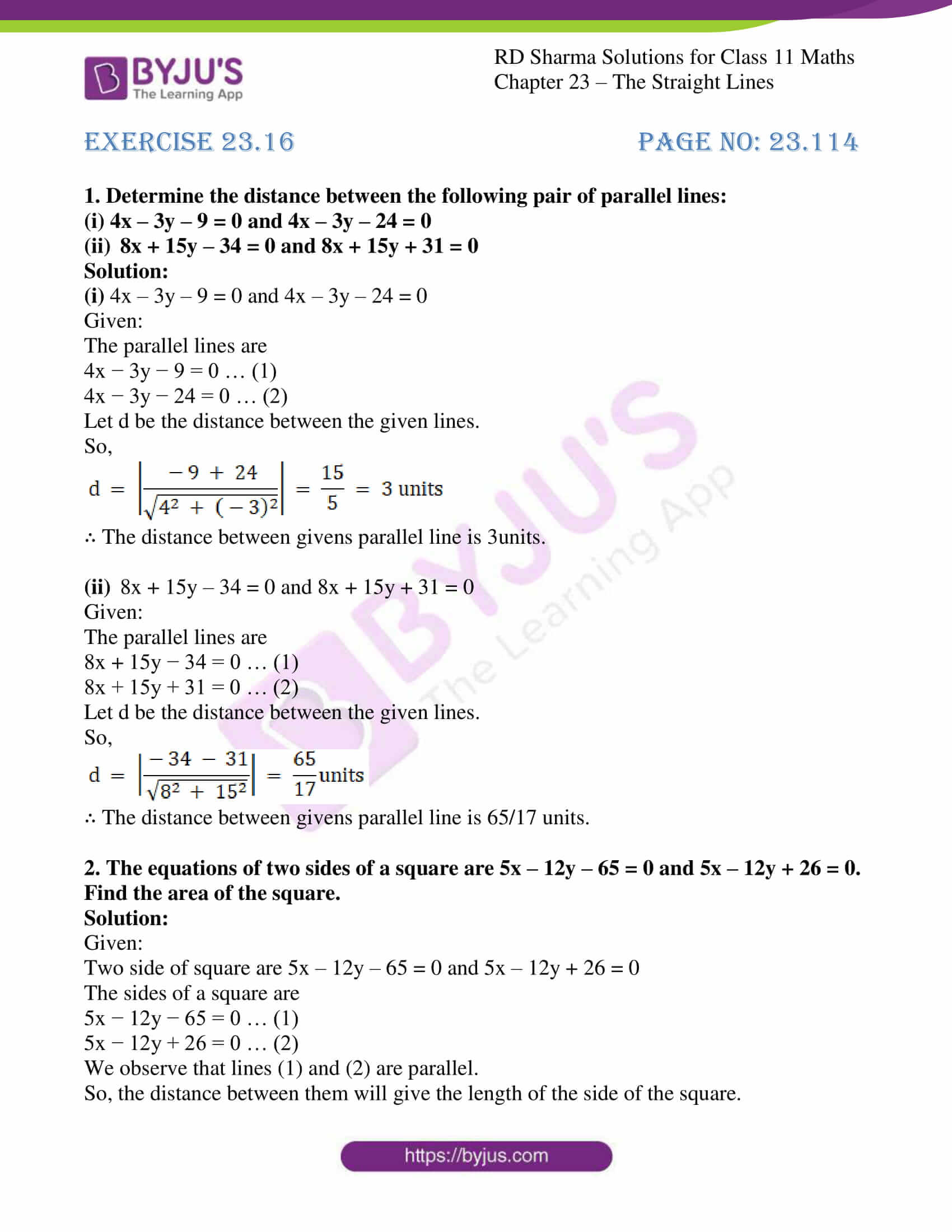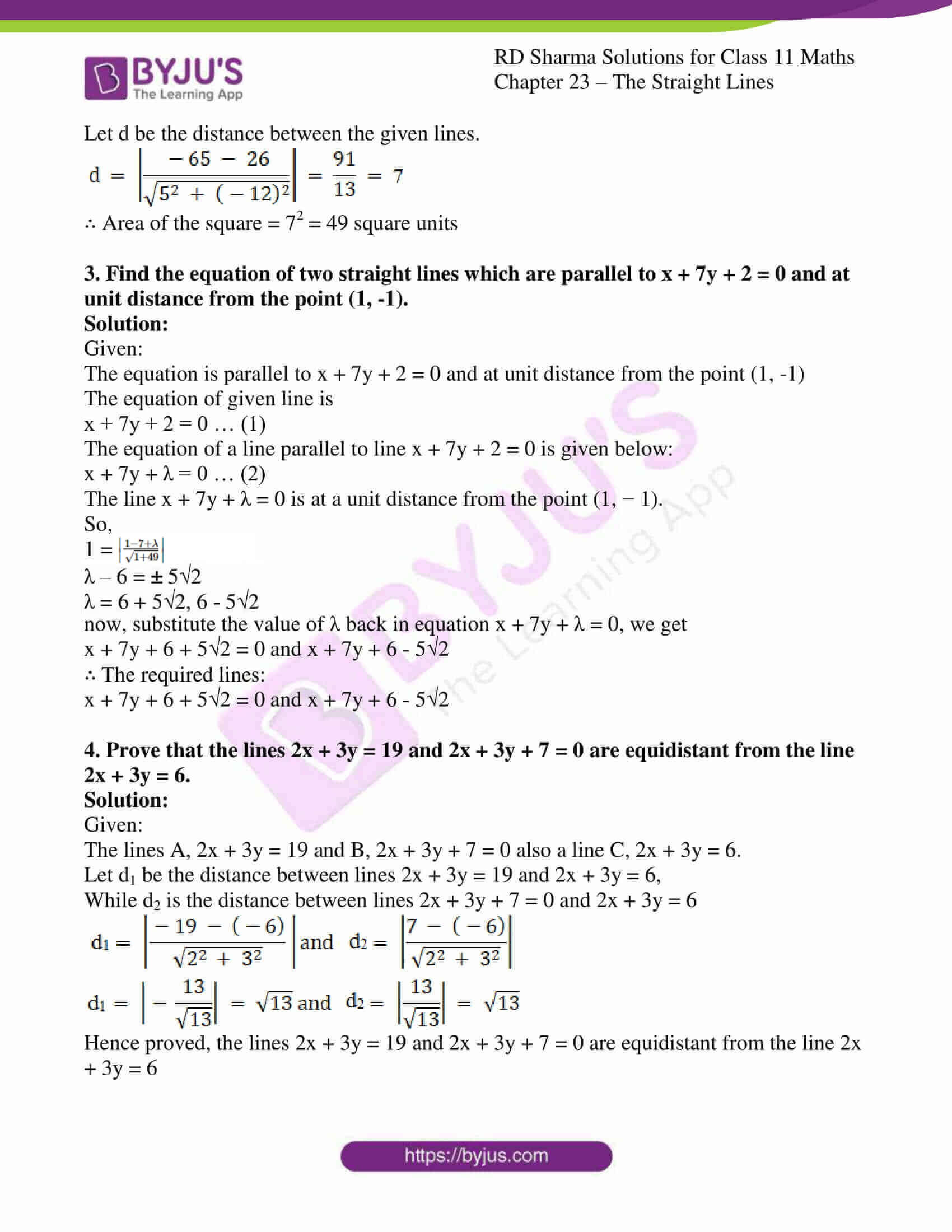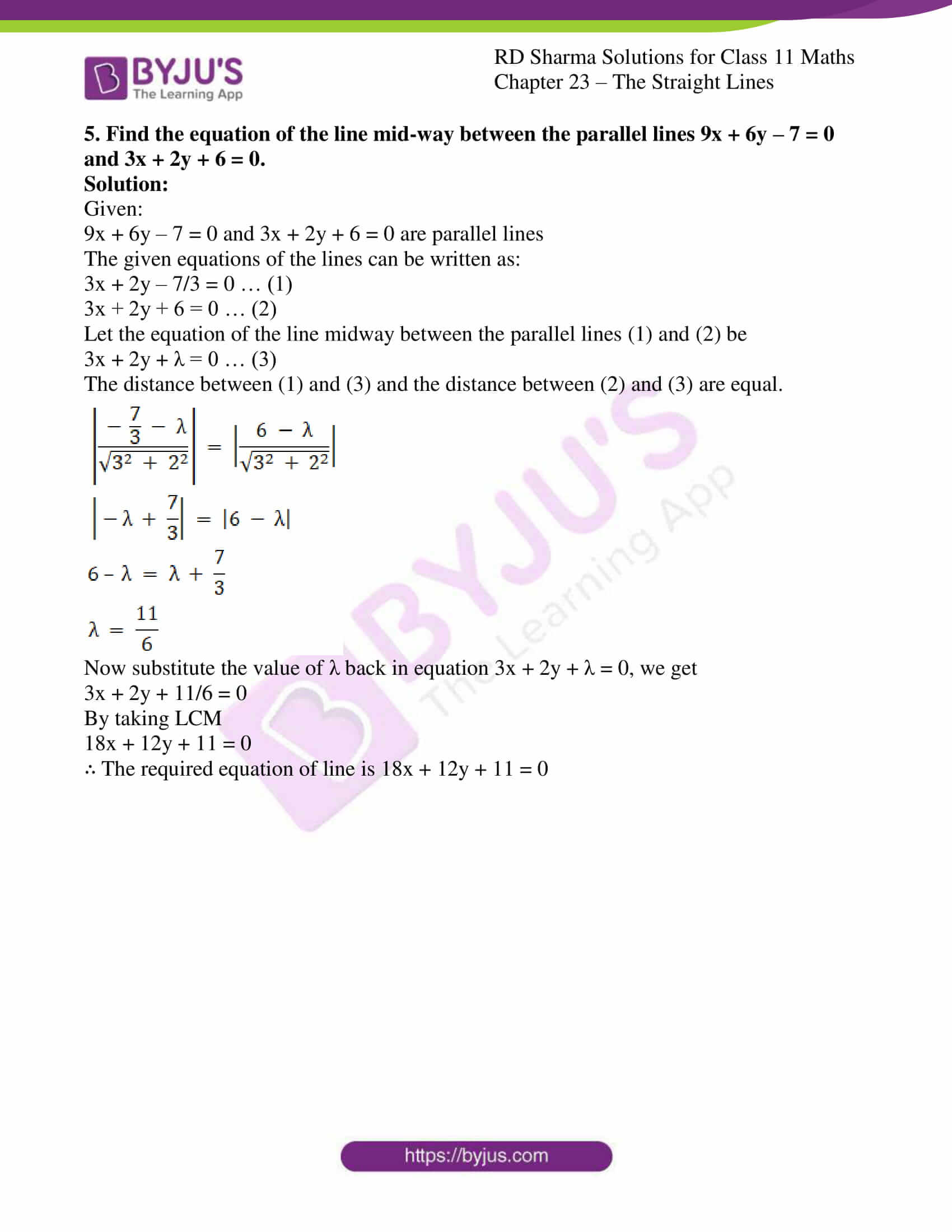### Also, access other exercises of RD Sharma Solutions for Class 11 Maths Chapter 23 – The Straight Lines

Exercise 23.1 Solutions

Exercise 23.2 Solutions

Exercise 23.3 Solutions

Exercise 23.4 Solutions

Exercise 23.5 Solutions

Exercise 23.6 Solutions

Exercise 23.7 Solutions

Exercise 23.8 Solutions

Exercise 23.9 Solutions

Exercise 23.10 Solutions

Exercise 23.11 Solutions

Exercise 23.12 Solutions

Exercise 23.13 Solutions

Exercise 23.14 Solutions

Exercise 23.15 Solutions

Exercise 23.17 Solutions

Exercise 23.18 Solutions

Exercise 23.19 Solutions

### Access answers to RD Sharma Solutions for Class 11 Maths Exercise 23.16 Chapter 23 – The Straight Lines

#### EXERCISE 23.16 PAGE NO: 23.114

1. Determine the distance between the following pair of parallel lines:
(i) 4x – 3y – 9 = 0 and 4x – 3y – 24 = 0

(ii) 8x + 15y – 34 = 0 and 8x + 15y + 31 = 0

Solution:

(i) 4x – 3y – 9 = 0 and 4x – 3y – 24 = 0

Given:

The parallel lines are

4x − 3y − 9 = 0 … (1)

4x − 3y − 24 = 0 … (2)

Let d be the distance between the given lines.

So,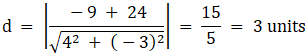∴ The distance between givens parallel line is 3units.

(ii) 8x + 15y – 34 = 0 and 8x + 15y + 31 = 0

Given:

The parallel lines are

8x + 15y − 34 = 0 … (1)

8x + 15y + 31 = 0 … (2)

Let d be the distance between the given lines.

So,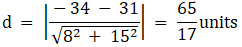∴ The distance between givens parallel line is 65/17 units.

2. The equations of two sides of a square are 5x – 12y – 65 = 0 and 5x – 12y + 26 = 0. Find the area of the square.

Solution:

Given:

Two side of square are 5x – 12y – 65 = 0 and 5x – 12y + 26 = 0

The sides of a square are

5x − 12y − 65 = 0 … (1)

5x − 12y + 26 = 0 … (2)

We observe that lines (1) and (2) are parallel.

So, the distance between them will give the length of the side of the square.

Let d be the distance between the given lines.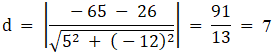∴ Area of the square = 72 = 49 square units

3. Find the equation of two straight lines which are parallel to x + 7y + 2 = 0 and at unit distance from the point (1, -1).

Solution:

Given:

The equation is parallel to x + 7y + 2 = 0 and at unit distance from the point (1, -1)

The equation of given line is

x + 7y + 2 = 0 … (1)

The equation of a line parallel to line x + 7y + 2 = 0 is given below:

x + 7y + λ = 0 … (2)

The line x + 7y + λ = 0 is at a unit distance from the point (1, − 1).

So,

1 =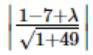λ – 6 = ± 52

λ = 6 + 52, 6 – 52

now, substitute the value of λ back in equation x + 7y + λ = 0, we get

x + 7y + 6 + 52 = 0 and x + 7y + 6 – 52

∴ The required lines:

x + 7y + 6 + 52 = 0 and x + 7y + 6 – 52

4. Prove that the lines 2x + 3y = 19 and 2x + 3y + 7 = 0 are equidistant from the line 2x + 3y = 6.

Solution:

Given:

The lines A, 2x + 3y = 19 and B, 2x + 3y + 7 = 0 also a line C, 2x + 3y = 6.

Let d1 be the distance between lines 2x + 3y = 19 and 2x + 3y = 6,

While d2 is the distance between lines 2x + 3y + 7 = 0 and 2x + 3y = 6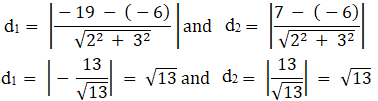Hence proved, the lines 2x + 3y = 19 and 2x + 3y + 7 = 0 are equidistant from the line 2x + 3y = 6

5. Find the equation of the line mid-way between the parallel lines 9x + 6y – 7 = 0 and 3x + 2y + 6 = 0.

Solution:

Given:

9x + 6y – 7 = 0 and 3x + 2y + 6 = 0 are parallel lines

The given equations of the lines can be written as:

3x + 2y – 7/3 = 0 … (1)

3x + 2y + 6 = 0 … (2)

Let the equation of the line midway between the parallel lines (1) and (2) be

3x + 2y + λ = 0 … (3)

The distance between (1) and (3) and the distance between (2) and (3) are equal.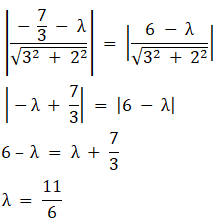Now substitute the value of λ back in equation 3x + 2y + λ = 0, we get

3x + 2y + 11/6 = 0

By taking LCM

18x + 12y + 11 = 0

∴ The required equation of line is 18x + 12y + 11 = 0